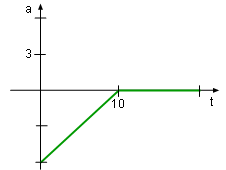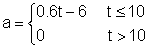Ch 6. Integrals Multimedia Engineering Math IndefiniteIntegral Area DefiniteIntegral FundamentalTheorem SubstitutionRule
 Chapter 1. Limits 2. Derivatives I 3. Derivatives II 4. Mean Value 5. Curve Sketching 6. Integrals 7. Inverse Functions 8. Integration Tech. 9. Integrate App. 10. Parametric Eqs. 11. Polar Coord. 12. Series Appendix Basic Math Units Search eBooks Dynamics Fluids Math Mechanics Statics Thermodynamics Author(s): Hengzhong Wen Chean Chin Ngo Meirong Huang Kurt Gramoll ©Kurt GramollMATHEMATICS - CASE STUDY IntroductionAcceleration of a Typical Raindrop Over Time As raindrops fall, their surface enlarges which increases the resistance to accelerate. This acceleration can be modeled aswhere t is time. Assume the rain starts 800 meters above the ground and has an initial velocity of 10 m/s. Questions How long does it take the raindrop to reach the ground? Approach The velocity is the antiderivative (integral) of the acceleration. The distance is the antiderivative (integral) of the velocity.